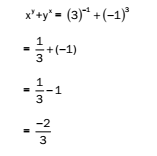# RRB ALP 2018 Practice Test Papers | Arithmetic Questions (Day-17)

Dear Aspirants, Here we have given the Important RRB ALP & Technicians Exam 2018 Practice Test Papers. Candidates those who are preparing for RRB ALP 2018 can practice these Arithmetic Questions to get more confidence to Crack RRB 2018 Examination.

[WpProQuiz 1676]

### Click “Start Quiz” to attend these Questions and view Explanation

1. The average of first thirty natural numbers is
1. 13.5
2. 14.5
3. 15.5
4. 16.5
1. A man borrows Rs. 5,000 and pays back after 4 years at 15% simple interest. The amount paid by the man is
1. Rs. 9,000
2. Rs. 8,000
3. Rs. 7,800
4. Rs. 8,400
1. If a @ b = a2x b2 + ab , then 3 @ 8 =
1. 600
2. 552
3. 576
4. 625
1. Find the greatest three-digit number that is exactly divisible by 15, 25, 20 and 30.
1. 990
2. 900
3. 960
4. 930
1. Two students Ria and Yana were paid a total amount of Rs. 840 per week by their parents. If Ria was paid 140% of the sum paid to Yana, then calculate the amount paid to Yana per week.
1. 450
2. 550
3. 675
4. 350
1. If x = 3 and y = -1, find the value of xy + yx.
1. 1/3
2. – 1/3
3. 2/3
4. – 2/3
1. A polygon has 35 diagonals. The number of sides in this polygon is
1. 13
2. 12
3. 11
4. 10
1. Akash purchased 4 articles for Rs. 140 and sold 5 articles for Rs. 200. His profit percentage is
1. 12.25%
2. 18.80%
3. 14.28%
4. 24%
1. What is the (01101)2 – (11000)2 =?
1. 10101
2. 11001
3. 10111
4. 10100
1. The number obtained by interchanging the digits of a two-digit number is less than the original number by 18, and the sum of the digits is 6. What is the original two-digit number?
1. 46
2. 24
3. 42
4. 64

Solution:

Sum of first 30 natural numbers n(n+ 1) /2 =30(30+ 1)/ 2  = 465

Therefore, average = 465/30 = 15.5

S.I. = 5000x15x4/100 = Rs. 3,000

Amount = 5000 + 3000 = Rs. 8,000

3 @ 8 = 32 x  82+ (3 x8) = 9 x64 + 24 = 576 + 24 = 600

Greatest 3-digit number = 999

LCM of 15, 20, 25 and 30 = 300

On dividing 999 by 300, remainder = 99

Hence, the required number = 999 – 99 = 900

Consider the amount paid to Yana = x. A

mount paid to Ria = 140% of x = 1.4x

Now, x + 1.4x = 840

2.4x = 840

x = 350Number of diagonals in a polygon of n sides = n( n- 3) /2

n( n- 3) /2 = 35

n2 – 3n – 70 = 0

(n + 7)(n – 10) = 0

n = 10

LCM of 4 and 5 = 20 Now, C.P. of 4 articles = Rs. 140

C.P. of 20 articles = 140 × 5 = Rs. 700

S.P. of 5 articles = Rs. 200

S.P. of 20 articles = 200 × 4 = 800

Profit = 800 – 700 = 100

Profit percentage = 100/700*100  = 14.28%

Use 1’s complement method Calculation:

1’s compliment of 11000 = 00111

Finding the difference

01101

00111

——–

10100

1

———-

10101

———–

Hence, the value is 10101.

Let the required two-digit number be xy, i.e. 10x + y.

The number obtained by interchanging the digits is 10y + x.

According to the question, 10x + y – 10y – x

9x – 9y = 18

x – y = 2

x + y = 6 (Given)

On solving these equations, we get:

x = 4 and y = 2

So, the required number = 42

RRB ALP 2018 Practice Test Papers | Arithmetic Questions (Day-1)

RRB ALP 2018 Practice Test Papers | Arithmetic Questions (Day-2)

RRB ALP 2018 Practice Test Papers | Arithmetic Questions (Day-3)

RRB ALP 2018 Practice Test Papers | Arithmetic Questions (Day-4)

RRB ALP 2018 Practice Test Papers | Arithmetic Questions (Day-5)

RRB ALP 2018 Practice Test Papers | Arithmetic Questions (Day-6)

RRB ALP 2018 Practice Test Papers | Arithmetic Questions (Day-7)

RRB ALP 2018 Practice Test Papers | Arithmetic Questions (Day-8)

RRB ALP 2018 Practice Test Papers | Arithmetic Questions (Day-9)

RRB ALP 2018 Practice Test Papers | Arithmetic Questions (Day-10)

RRB ALP 2018 Practice Test Papers | Arithmetic Questions (Day-11)

RRB ALP 2018 Practice Test Papers | Arithmetic Questions (Day-12)

RRB ALP 2018 Practice Test Papers | Arithmetic Questions (Day-13)

RRB ALP 2018 Practice Test Papers | Arithmetic Questions (Day-14)

RRB ALP 2018 Practice Test Papers | Arithmetic Questions (Day-15)

RRB ALP 2018 Practice Test Papers | Arithmetic Questions (Day-16)